Next: Axially symmetric mass distributions Up: Newtonian gravity Previous: Gravitational potential

# Gravitational potential energy

Consider a collection of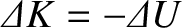point masses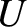located at position vectors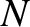(where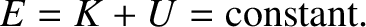runs from 1 to). What is the gravitational potential energy stored in such a collection? In other words, how much work would we have to do in order to assemble the masses, starting from an initial state in which they are all at rest and very widely separated?

We have seen that a gravitational acceleration field can be expressed in terms of a gravitational potential: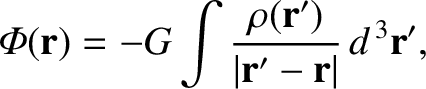(3.11)

We also know that the gravitational force acting on a masslocated at position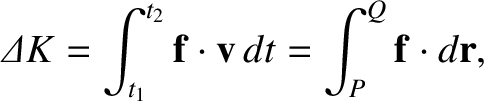is written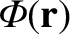(3.12)

The work we would have to do against the gravitational force to slowly move the mass from point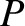to point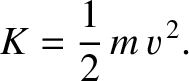is simply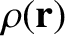(3.13)

The negative sign in the preceding expression comes about because we would have to exert a force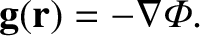on the mass to counteract the force exerted by the gravitational field. Recall, finally, that the gravitational potential generated by a point mass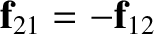located at position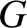is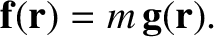(3.14)

Let us build up our collection of masses one by one. It takes no work to bring the first mass from infinity, because there is no gravitational field to fight against. Let us clamp this mass in position at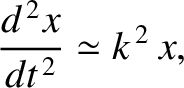. In order to bring the second mass into position at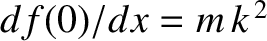, we have to do work against the gravitational field generated by the first mass. According to Equations (3.13) and (3.14), this work is given by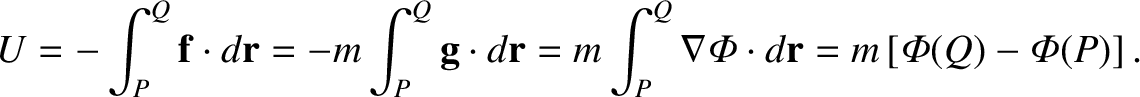(3.15)

Let us now bring the third mass into position. Because gravitational fields and gravitational potentials are superposable, the work done while moving the third mass from infinity to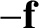is simply the sum of the works done against the gravitational fields generated by masses 1 and 2 taken in isolation: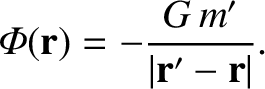(3.16)

Thus, the total work done in assembling the arrangement of three masses is given by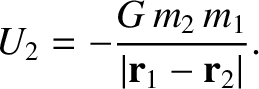(3.17)

This result can easily be generalized to an arrangement ofpoint masses, giving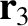(3.18)

The restriction that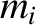must be less thanmakes the preceding summation rather messy. If we were to sum without restriction (other than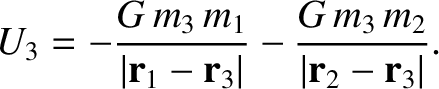) then each pair of masses would be counted twice. It is convenient to do just this, and then to divide the result by two. Thus, we obtain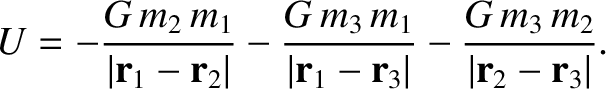(3.19)

This is the potential energy of an arrangement of point masses. We can think of this quantity as the work required to bring the masses from infinity and assemble them in the required formation. The fact that the work is negative implies that we would gain energy during this process.

Equation (3.19) can be written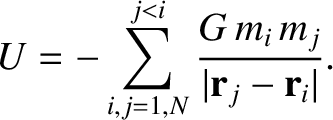(3.20)

where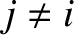(3.21)

is the gravitational potential experienced by theth mass due to the other masses in the distribution. For the case of a continuous mass distribution, we can generalize the preceding result to give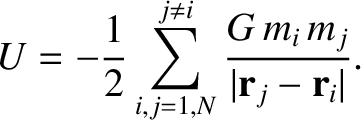(3.22)

where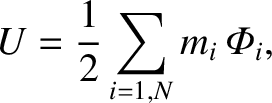(3.23)

is the familiar gravitational potential generated by a continuous mass distribution of mass density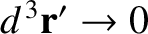.Next: Axially symmetric mass distributions Up: Newtonian gravity Previous: Gravitational potential
Richard Fitzpatrick 2016-03-31